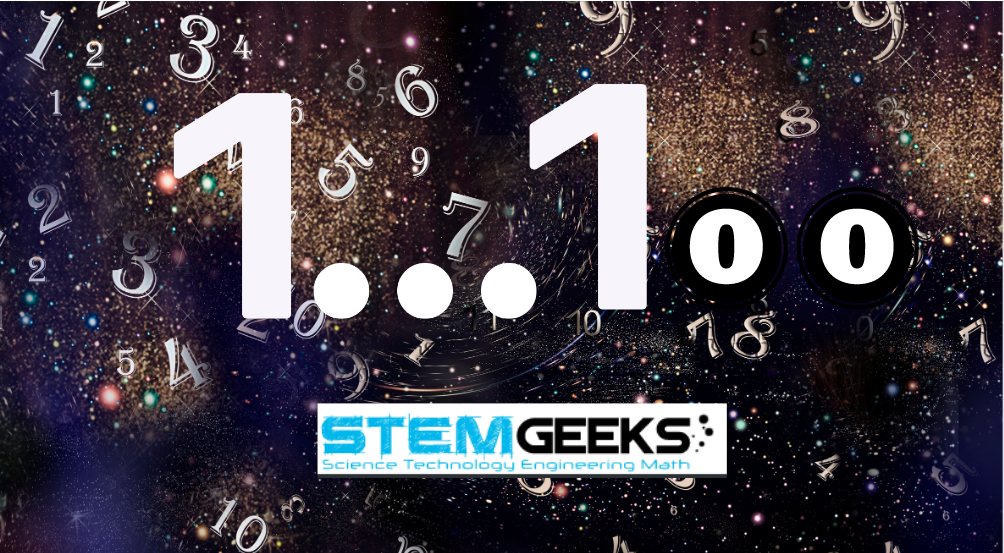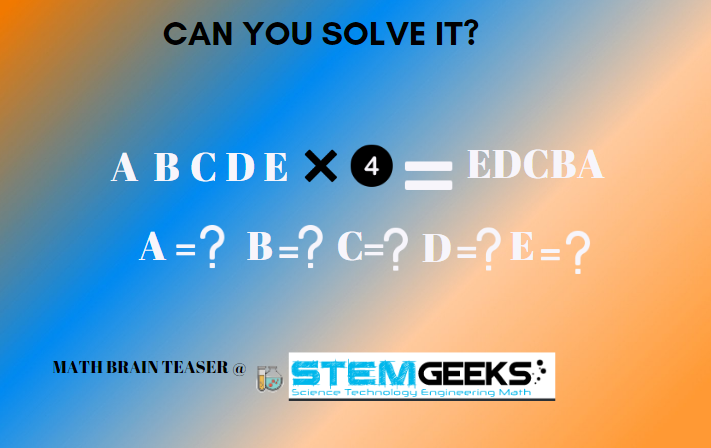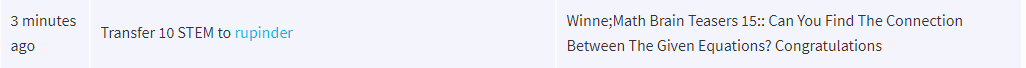# Math Brain Teasers 16:: Find How Many Times Number Puzzle?

in STEMGeeks10 months ago

Hey All; @StemGeeks Mathematician;

It's a weekend and are you ready to muscle your brain for today's' "Math Brain Teasers"? I guess the last one was a difficult one but this time I assure you that it's not going to be that complex. At times we do need to have complex puzzles to solve so that we are spending some time thinking to find the right answer and that's how you get better at anything you do.

With that let's get going with our Math Brain Teasers series question & answers. Have a close look at the given image and try finding out; How Many times do the number::

### 5 occur in the numbers from 1 to 100?Hint:: I guess this should be an easy one to solve. All that I can say is that be careful with your calculations and you should be able to arrive at the right answer. What you need to find is how many times does the number 5 occurs in the number range 1 to 100; keep this in mind while solving the puzzle.

##### Math Brain Teasers 15:: Can You Find The Connection Between The Given Equations? - Solved with ExplanationIn order to solve this maths puzzle, we need to think of a number when by 4, the opposite should appear. And as rightly stated by @rupinder in the comment that only 2 and 8 are the numbers which when multiplied by 4 can give 8 and 2 which would be a reversible number. Applying the same logic for the rest part of the equation the answer would be::

### This number is: 21978

Hence the answer to the puzzle is as follows::

### STEM token GiveAway

I'll be again doing a giveaway of STEM tokens to the lucky random winner with the correct answer. For the last contest, which was Can You Find The Connection Between The Given Equations?.

We had 2 entries & out of which 1 was correct. Congratulations @rupinder; You WIN yourself 10 STEM tokens. You should be having the rewards in your STEM Wallet Soon.### Math Quote for the Day::

Here is the motivation to solve this Number puzzle; How many numbers do you see??source::

If you like my work, then please spread the Word.. that we do have the Math Brain Teasers competition here @StemGeeks platform. Reblog is much appreciated.

Best RegardsFind Me on the Other Social Media Platforms::PS:: All the Maths Brain Teasers; are made by me using the Pro Canva License Version

Sort:

11

19

10 months ago (edited)

Thank you so much for the award. Today’s answer is 19

19

19